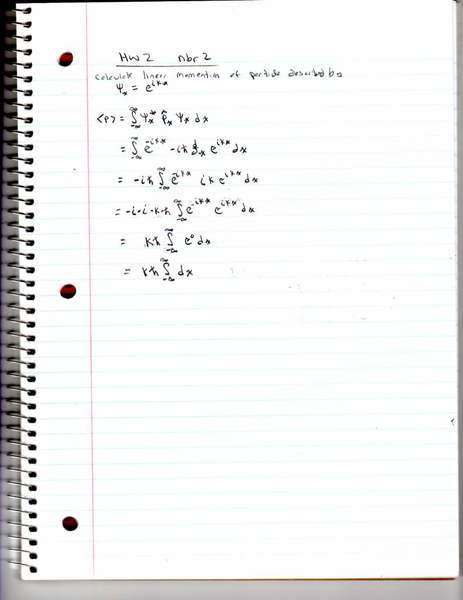# Linear momentum of particlethe attachment shows my work. I honestly think maybe i did something wrong. at any rate the integrand is 1 and so not really even or odd.

any help is appreciated

gabbagabbahey
Homework Helper
Gold Member
View attachment 51114

the attachment shows my work. I honestly think maybe i did something wrong. at any rate the integrand is 1 and so not really even or odd.

any help is appreciated

Where did the integral in your first step come from? Start with the definition of $\langle \hat{P} \rangle$...

Sorry, I am supposed to calculate the average linear momentum.
So would be <p> =∫ψ*(x)P(hat)ψ(x)dx

P(hat) = -ih(bar)(d/dx)

gabbagabbahey
Homework Helper
Gold Member
Sorry, I am supposed to calculate the average linear momentum.
So would be <p> =∫ψ*(x)P(hat)ψ(x)dx

P(hat) = -ih(bar)(d/dx)

The average linear momentum of what state?Remember, $\psi_k(x)=e^{ikx}$ is just one k-mode of the full wavefunction for a free particle $\psi(x)=\int_{-\infty}^{\infty} A(k) \psi_k(x)dk$.

Last edited:
gabbagabbahey
Homework Helper
Gold Member
View attachment 51122

I think I figured it out. Let me know if it looks correct. thanks

Does $\frac{\infty}{\infty}=1$? That is essentially what you are claiming in your last step.

As for your first step, is that the definition of average momentum you are using in your course? Usually one defines the expectation value, or average, of an operator $\hat{A}$ in a given state $|\psi\rangle$ as $\langle \hat{A} \rangle \equiv \langle \psi |\hat{A}|\psi \rangle$.

Frankly, I'm still not 100% clear on what the original problem is. Can you post the original problem verbatim (word for word)?

Just evaluate the integral [;\int_{-\infty}^{\infty} dx;]. What do you get?
Also, [; <p>= m\frac{d<x>}{dt} ;], so if you can find [;<x>;], you should also be able to find [;<p>;].